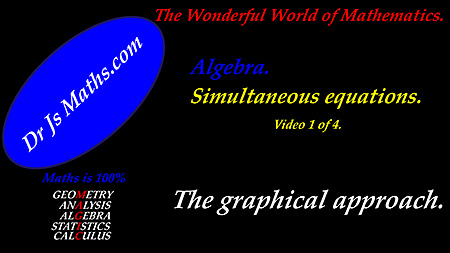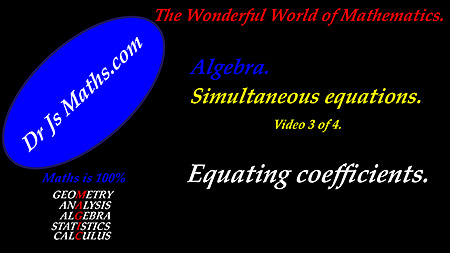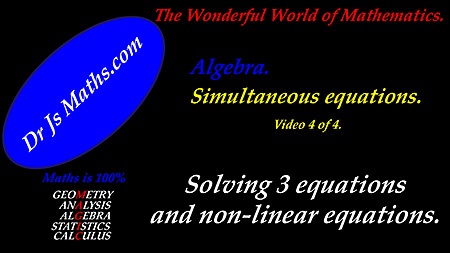Dr. J's Maths.com
Where the techniques of Maths
are explained in simple terms.

Algebra - Equations - Simultaneous equations.
Main page.

Solving simultaneous equations is the algebraic technique for finding values for variables which simultaneously satisfy conditions described by a set of equations.

The technique is very important in many fields of Mathematics.

The following resources can be accessed: Graphing

 Learning area Resource 1. Simultaneous equations - the graphical approach.Graphing simultaneous equations - Test Yourself 1. Graphing simultaneous equations - Solutions for Test Yourself 1. 2. Coefficient of one variable = 1 or coefficients of one variable equal.Easy coefficients - Test Yourself 1. Easy coefficients - Test Yourself 1 - Solutions. Easy coefficients - Test Yourself 2. Easy coefficients - Test Yourself 2 - Solutions. 3. Equating coefficients to solve simultaneous equations.Solving simultaneous equations by equating coefficients - Test Yourself 1. Solving simultaneous equations by equating coefficients - Solutions for Test Yourself 1. 4. Solving sets incorporating non-linear simultaneous equations.Solving non-linear simultaneous equations - Test Yourself 1. Solving simultaneous equations - Solutions for Test Yourself 1. 5. Solving sets of simultaneous equations with three equations. Solving sets of 3 equations - Test Yourself 1. Solving sets of 3 equations - Solutions to Test Yourself 1.